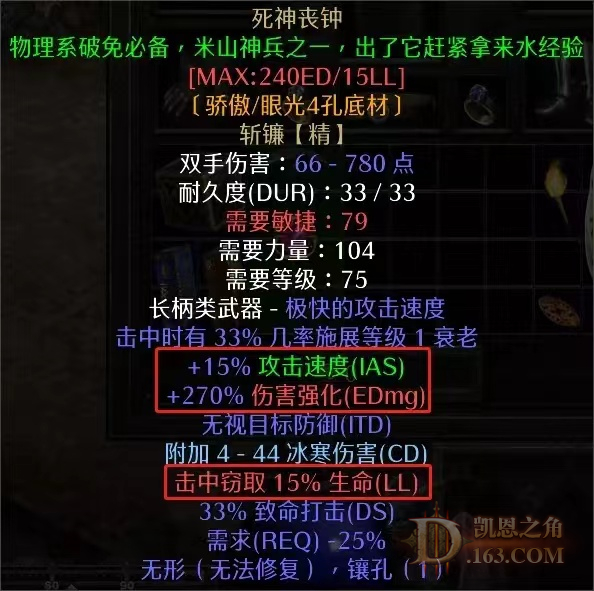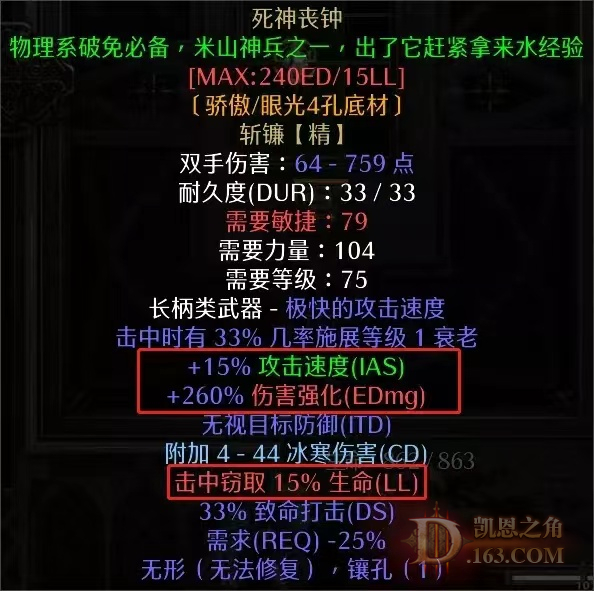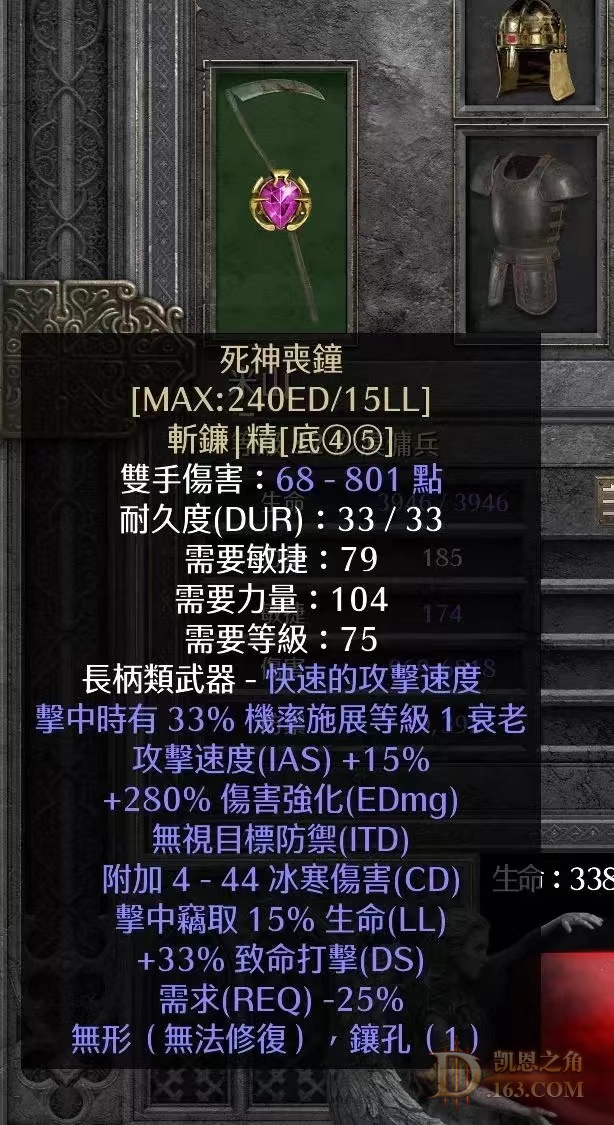//没登陆

 数据库是240，但是看到有260  270  280的 yyyJon#31266
SOR：塔拉夏新星电   SOR：手持无限新星电
SOR：纯冰全MF        SOR：44QH/火蛇
AMA：标马               BAR：55BO/打钱
NEC：塔格奥纯召
 in了猪，官服mod不知道有没有按“J”这个功能。

 带上镶嵌物最高290ed

 本帖最后由 不想肝了 于 2023-3-17 11:42 编辑 镶嵌27# 最高290

wlwsor#3155
S0：四神=0  符文全收集完成
24=2 25=7 26=2 27=2 28=1 29=3 30=1
31=2 32=1 33=1
S1\rune：32=1 31=2 30=1 29=2  28=1
27=2 25=3 24=4
Item：15/15 mp+ 200/9 wxtt 3/19/20 sc
S2\AFK
S3\rune：24=2 28=2 29=2 31=1
item: 451pd sc
S4\rune:  26=1